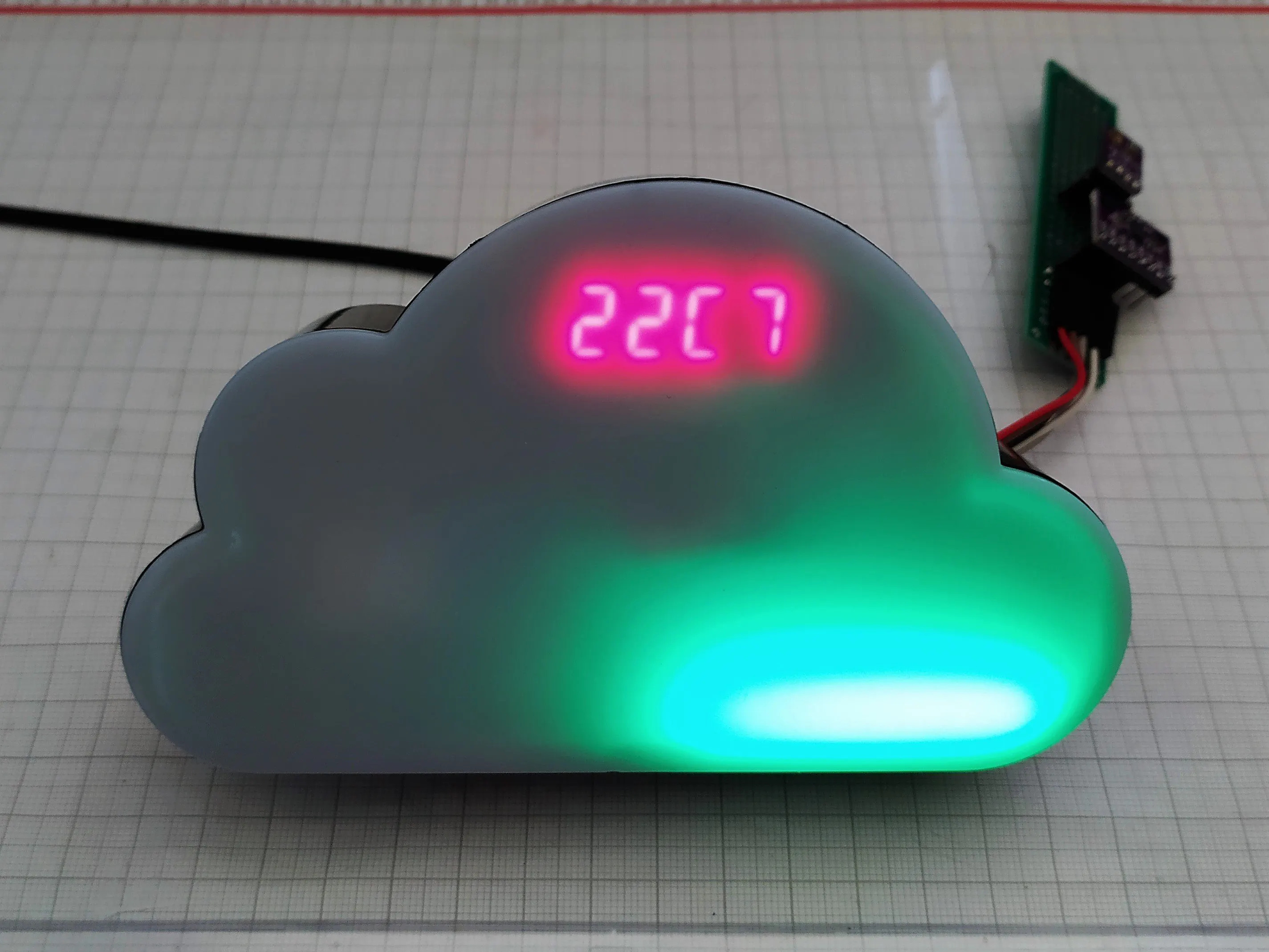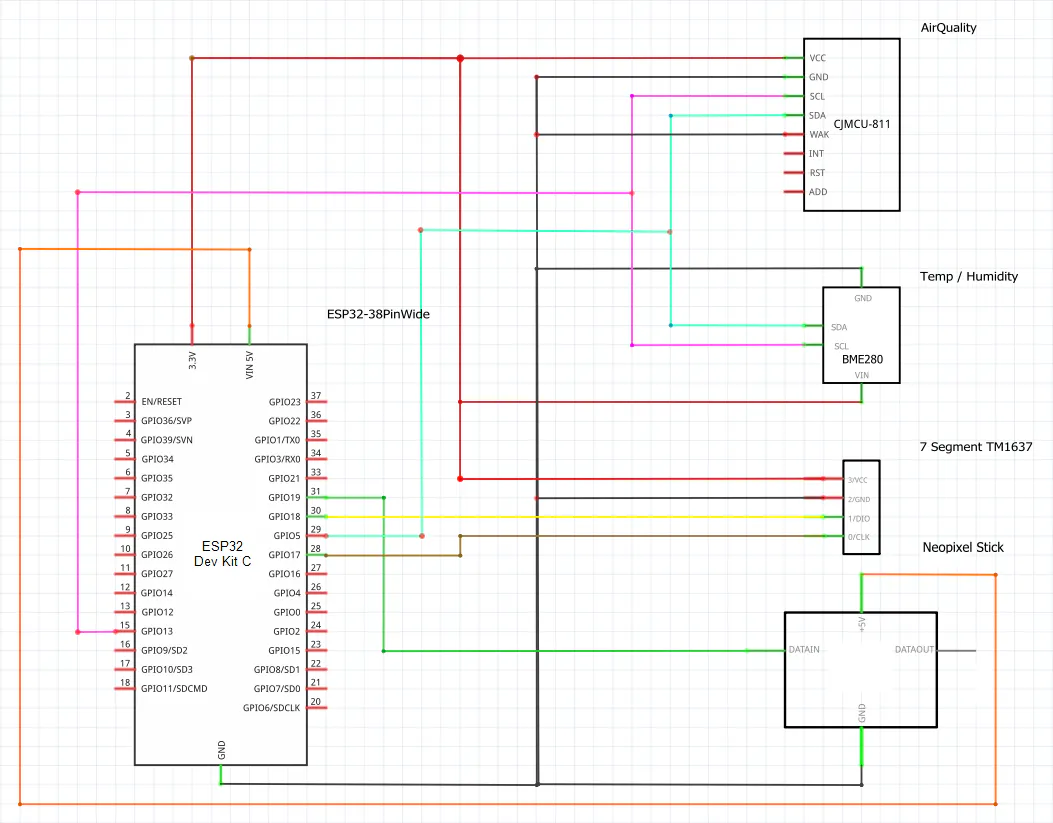# Indoor-air-quality-traffic-light

Indoor air quality traffic light with display and Mqtt support ( ESP32, BME280, CCS811, TM1637, Neopixel, Mqtt) with Micropython

IntermediateProtip3 hours812## Things used in this project

### Hardware components

 ESP 32 Dev Kit C
×1LED Stick, NeoPixel Stick
×1
 BME280 Temp Sensor
×1
 7 Segment Display with tm 1637
×1
 CJMCU CCS811 sensor
×1
 PCB Boards
×1
 Case Led Lightbox
×1

## Schematics

### Schematic

connect the parts## Code

### main.py

MicroPython
```from machine import Pin ,I2C , WDT , reset
from umqtt.simple import MQTTClient
import ubinascii
import neopixel
import tm1637
import time
import network
import CCS811
import bme280

####config start#######

#config Wifi
wifi_enable = False  #True or False for using Wifi

#config MQTT
mqtt_client = "yourMqttClientName"
mqtt_broker = "yourMqttBrokerIP"
mqtt_user = "yourMqttUser"
mqtt_port = 1883

#def MQTT  Topic
topic_co2 = b"home/livingroom/co2"
topic_tvoc = b"home/livingroom/tvoc"
topic_temp =  b"home/livingroom/temperature"
topic_humidity =  b"home/livingroom/humidity"
topic_press = b"home/livingroom/pressure"

####config end#######

#init Watchdog 10 sec
wdt = WDT(timeout=60000)
print("init sw watchdog ")

#NeoPixel def
pin = Pin(19,Pin.OUT)
np = neopixel.NeoPixel(pin, 8)

#7Segment def
tm = tm1637.TM1637(clk=Pin(17), dio=Pin(18))

#CCS811 def
i2c = I2C(scl=Pin(13), sda=Pin(5))

#BME280 def
bme = bme280.BME280(i2c=i2c)

# clear NeoPixel
def np_clear():
for i in range(8):
np[i] = (0, 0, 0)
np.write()

np_clear()  # clear

#def state for first run
state = 0

#def for use wifi
def connect_wifi():

sta_if = network.WLAN(network.STA_IF)
sta_if.active(True)
if not sta_if.isconnected():
print("connecting to wlan...")
while not sta_if.isconnected():
time.sleep(10)
pass
print(sta_if.ifconfig())

def check_wifi():
sta_if = network.WLAN(network.STA_IF)
if sta_if.isconnected() == True:
return True
else:
return False

def restart_and_reconnect():
try:
print("failed to connect to MQTT broker . Reconnecting...")
time.sleep(10)
reset()
except OSError as e:
restart_and_reconnect()

#create MQTT client

def check_co2():
try:
data_eCO2_tVOC = [s.eCO2, s.tVOC]
return data_eCO2_tVOC
time.sleep(1)

except:

def sub_neopixel_room_ambient(eCO2 , tVOC):
global state
co2_value = int(eCO2)
print("eCO2_value: %s , tVOC_value: %s " % (eCO2,tVOC) )

if co2_value  >= 1500:
#print("open the windows!")
for i in range(8):
np[i] = (16,0,0)
np.write()
state = 1
elif co2_value >= 900:
#print("mid air quailty")
for i in range(8):
np[i] = (32,16,0)
#yellow
np.write()
state = 1
elif co2_value < 900:
#print("good air quality")
for i in range(8):
np[i] = (0,16,0)
#green
np.write()
#tm.show(str(co2_value))
state = 1

elif state == 0:
print("no data aviable")
n=np.n
# bounce
for i in range(2 * n):
for j in range(n):
np[j] = (8, 0, 16)
if (i // n) % 2 == 0:
np[i % n] = (0, 0, 0)
else:
np[n - 1 - (i % n)] = (0, 0, 0)
np.write()
time.sleep_ms(60)

def  sub_display_7digit( temp):   #display data on 7Segment
print("Bme280 Temp: %s " % temp)
temp_value = temp
temp_value = str(temp_value).replace(".","C")
tm.show(temp_value)
#print((temp_value))

# let's go

if state == 0:
tm.show("Test")

n=np.n
# bounce for test neopixel
for i in range(2 * n):
for j in range(n):
np[j] = (0, 0, 8)
if (i // n) % 2 == 0:
np[i % n] = (0, 0, 0)
else:
np[n - 1 - (i % n)] = (0, 0, 0)
np.write()
time.sleep_ms(30)

connect_wifi()

measure_time = time.ticks_ms()
send_data_time = time.ticks_ms()

while 1:

try:
#15 min = 900000 millisec
#1 min = 60000 millisec
delta_measure = time.ticks_diff(time.ticks_ms(), measure_time)

delta_send_data = time.ticks_diff(time.ticks_ms(), send_data_time)

if delta_measure >= 60000 or state == 0: # 1 min = 60000 millisec or if state == 0  while first run then check the sensors

#measure bme280
t = r/100
t = t - 2  #offset TempSensor
p = r/25600
h = r/1024

s.put_envdata(humidity=h,temp=t)

#measure ccs811
air_data = check_co2()

if air_data is not None  :
# neopixel write
sub_neopixel_room_ambient(air_data,air_data)

# 7 digit write
sub_display_7digit(t)

measure_time = time.ticks_ms()

if wifi_enable == True:

if check_wifi() == True and delta_send_data >= 900000 :   # 15 min = 900000 millisec
try:
client.connect()
client.publish(topic_co2, str(air_data))
time.sleep(0.5)
client.publish(topic_tvoc, str(air_data))
time.sleep(0.5)
client.publish(topic_temp, str(t))
time.sleep(0.5)
client.publish(topic_humidity,str(h))
time.sleep(0.5)
client.publish(topic_press,str(p))
time.sleep(0.5)

send_data_time = time.ticks_ms()
client.disconnect()

except:
restart_and_reconnect()

elif delta_send_data >= 900000:

send_data_time = time.ticks_ms()

else :
pass

wdt.feed()

except OSError as e:
print("Error while loop")

```

## Credits

### DAN

3 projects • 1 follower